# Weak homotopy equivalence of topological spaces

This article defines a property of continuous maps between topological spaces

## Definition

### Definition for path-connected spaces in terms of homotopy groups

Let$A$ and$B$ be path-connected spaces. A weak homotopy equivalence from$A$ to$B$ is a continuous map$f:A \to B$ such that the functorially induced maps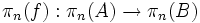$\pi_n(f):\pi_n(A) \to \pi_n(B)$ are group isomorphisms for all$n \ge 1$.

Note that since the maps are homomorphisms anyway, it is enough to require them to be bijective.

Basepoint choice disclaimer for homotopy group isomorphism: To concretely define the map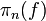$\pi_n(f)$, we need to choose basepoints for$A$ and$B$. Change of basepoint, however, only results in pre-composition and post-composition by isomorphisms and does not affect whether or not the map is an isomorphism.

### Equivalent definition for path-connected spaces in terms of homology groups

Let$A$ and$B$ be path-connected spaces. Then a continuous map$f:A \to B$ is a weak homotopy equivalence iff both of these conditions hold:

• The induced map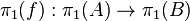$\pi_1(f):\pi_1(A) \to \pi_1(B)$ is an isomorphism of groups.
• For every bundle of abelian groups$\mathcal A$ over$B$, the induced map of twisted homology groups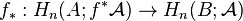$f_*:H_n(A;f^* \mathcal A) \to H_n(B;\mathcal A)$ is an isomorphism of groups for all$n \ge 0$.

As above, all of these maps are homomorphisms anyway, so it is enough to require them to be bijective. The above basepoint disclaimer for$\pi_1$ also applies here.

If both$A$ and$B$ are simply connected then the criterion is simpler: a continuous map$f:A \to B$ is a weak homotopy equivalence iff the induced map on homology with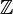$\mathbb Z$ coefficients$f_*:H_n(A; \mathbb Z) \to H_n(B; \mathbb Z)$ is an isomorphism of groups for all$n \ge 0$.

### Definition for spaces that are not path-connected

Let$A$ and$B$ be topological spaces. A weak homotopy equivalence from$A$ to$B$ is a continuous map$f:A \to B$ such that:

• The functorially induced map$\pi_0(f): \pi_0(A) \to \pi_0(B)$ is a bijection between the set of path components$\pi_0(A)$ and the set of path components$\pi_0(B)$.
• For every path component of$A$, the restriction of$f$ to a continuous map from that to its image path component of$B$ is a weak homotopy equivalence of path-connected spaces.

## Facts

• The existence of a weak homotopy equivalence from$A$ to$B$ does not imply the existence of a weak homotopy equivalence from$B$ to$A$. Thus, to get an equivalence relation on topological spaces, we need to take a symmetric transitive closure. We say that spaces are weak homotopy-equivalent topological spaces if they are in the same equivalence class under the equivalence relation thus obtained.
• The mere fact that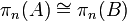$\pi_n(A) \cong \pi_n(B)$ as abstract groups is not enough to guarantee that$A$ and$B$ are weak homotopy-equivalent, even when$A$ and$B$ are manifolds or CW-spaces (see isomorphic homotopy groups not implies weak homotopy-equivalent). Rather, it is specifically important that the map must induce those isomorphisms.
• The exception to the above is in the case that both$\pi_n(A)$ and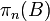$\pi_n(B)$ are the trivial group/one-point set for all$n$. In this case, any map must induce isomorphisms since that's the only possible map between trivial groups/one-point sets. In this case, the spaces$A$ and$B$ are both weakly contractible spaces.
• Similarly, the mere fact that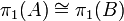$\pi_1(A) \cong \pi_1(B)$ as abstract groups and$H_n(A) \cong H_n(B)$ as abstract groups does not imply that$A$ and$B$ are weak homotopy-equivalent. See isomorphic homology groups and isomorphic fundamental groups not implies weak homotopy-equivalent. Rather, it is specifically important that the map must induce those isomorphisms.
• The exception to the above is, once again, where the fundamental group and all the homology groups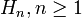$H_n, n \ge 1$, are trivial.